Chapter Notes: Work and Energy

# Work and Energy Chapter Notes - Science Class 9

Studying Work, Power, and Energy is crucial as it helps us understand the fundamental principles governing the conversion and transfer of energy in various systems. This knowledge is essential for designing efficient machines, optimizing industrial processes, and developing sustainable energy solutions.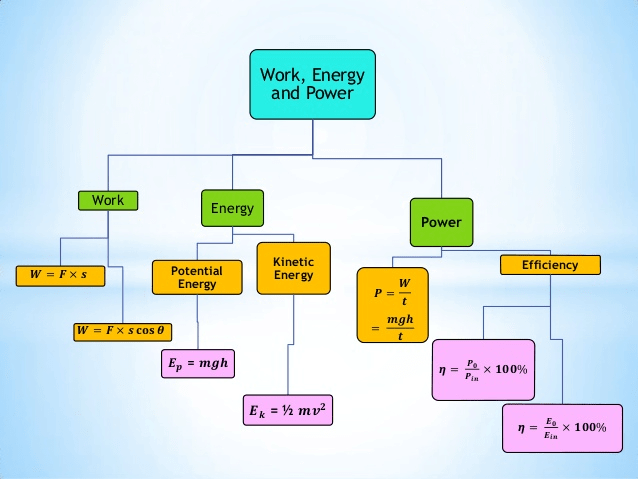Brief overview of Work, Power and Energy

## What is Work?

Work is defined as the product of the constant force applied on an object and the displacement of the object in the direction of the applied force.

For work to be done, two essential conditions must be met:

(i) the application of a force on the object, and

(ii) the displacement of the object in the direction of the force.

Mathematically, work (W) can be calculated as :

W = F x s,

where F represents the constant force applied and s represents the displacement along the direction of the force.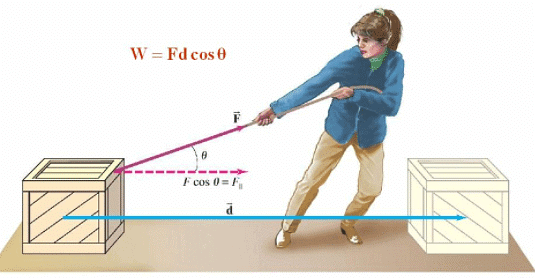Work Done

• SI unit of work is called joule (J or Nm). Work is said to be 1 joule if under the influence of a force of 1 N the object moves through a distance of 1 m along the direction of applied force.
• Work has only a magnitude and no sense of direction i.e., it is a scalar.

Example: A force of 10 Newtons is applied to an object, causing it to be displaced by 5 meters. What is the work done on the object?

Solution: We can use the equation W = F x s, where:

W represents the work done,

F represents the force applied,

s represents the displacement.

Given:

F = 10 Newtons

s = 5 meters

Plugging these values into the equation, we have:

W = 10 N x 5 m

W = 50 Joules

Therefore, the work done on the object is 50 Joules.

What is Positive & Negative Work Done?Work is considered positive if the displacement of the object is along the direction of force applied. On the other hand, work is taken as negative if the displacement of the object is in a direction opposite to the direction of force applied.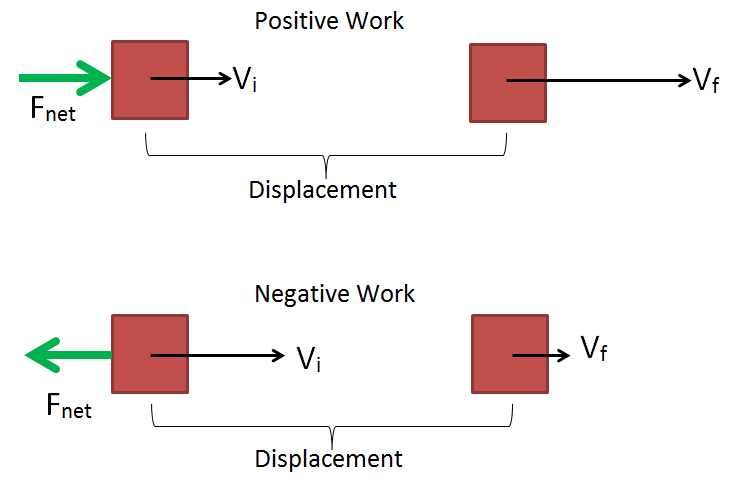Positive and Negative Work Done

Example: Work done by a man is taken positive when he moves from ground floor to second floor of his house. But work done by the same man is negative when he is descending from second floor of house to ground floor.

What is Zero Work Done?

If displacement of an object is in a direction perpendicular to the application of force, work done is zero inspite of the fact that force is acting and there is some displacement too.

Example: Imagine a person pushing a lawn roller across a lawn. The person applies a force horizontally to push the roller, while the displacement of the roller is also horizontal. Meanwhile, the force of gravity acts vertically downwards on the roller, but this force does not contribute to the work done on the roller, as it is perpendicular to the direction of displacement. Therefore, the work done by gravity in this case is zero, even though there is a force acting and displacement occurring.

Question for Chapter Notes: Work and Energy
Try yourself:Question 1: The work done on an object does not depend upon the

### Not Much ‘work’ In Spite of Working Hard!

In everyday life, we often use the term "work" to describe activities that involve physical or mental effort. However, according to the scientific definition of work, it may not always align with our common understanding.

Example: in the context of pushing a rock, even if we exert a lot of effort and get exhausted, if the rock doesn't move, no work is done according to the scientific definition.

### Scientific Conception of Work

Scientifically, work is defined as the application of force on an object resulting in its displacement. Let's consider a few situations to better understand this concept:

• Pushing a pebble: When you push a pebble and it moves a certain distance, you have exerted force on the pebble and it has been displaced. Therefore, work is done in this situation.
• Pulling a trolley: If a girl pulls a trolley and it moves, both the force applied by the girl and the displacement of the trolley meet the conditions for work. Hence, work is done in this scenario.
• Lifting a book: When you lift a book by applying a force, the book moves upwards, satisfying both conditions for work. Therefore, work is done in this case.

### Work Done By A Constant Force

Work done by a constant force can be calculated using the formula:

Work = Force × Distance × cos(θ)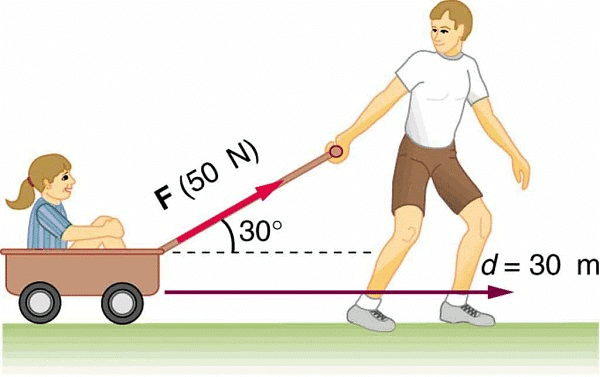Work Done

where:

• Force is the magnitude of the constant force applied
• Distance is the displacement of the object in the direction of the force
• θ is the angle between the force vector and the displacement vector
• If the force and displacement are in the same direction (θ = 0), the formula simplifies to:

Work = Force × Distance

• This indicates that work done by a constant force is equal to the product of the force applied and the distance over which the force acts.
• It's important to note that work is a scalar quantity, meaning it has magnitude but no direction. The units of work are joules (J) in the International System of Units (SI).

## What is Energy?

An object having the capability to do work is said to possess energy. Hence, the energy of an object is defined as its capacity of doing work. Energy of an object is measured by the total amount of work done by the object.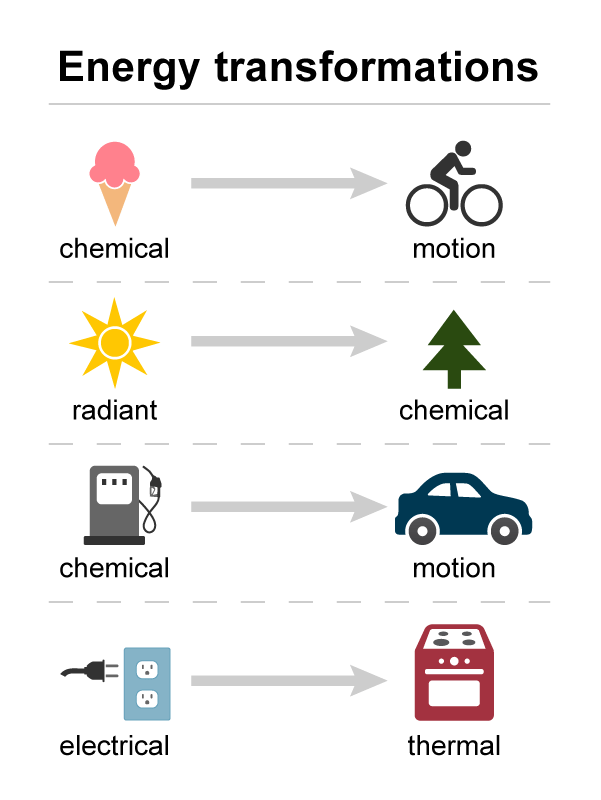Energy Transformation

Unit of energy is same as the unit of work. So, SI unit of energy will be joule (J). Energy too has magnitude only.

### Forms of Energy

Energy has many forms. Some important forms of energy are mechanical energy, heat energy, electrical energy, light energy, chemical energy, nuclear energy etc.

Mechanical energy is of two kinds, namely,

(i) Kinetic energy

(ii) Potential energy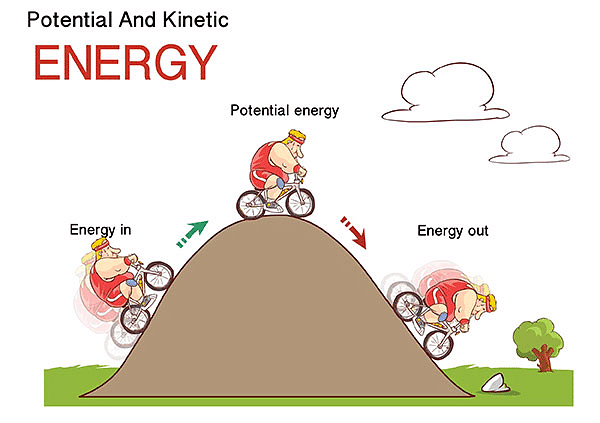Potential and Kinetic Energy

### Kinetic Energy

Kinetic Energy of an object is the energy possessed by it by virtue of its state of motion. A speeding vehicle, a rolling stone, a flying aircraft, flowing water, blowing wind, a running athlete possess kinetic energy.

For an object of mass m and having a speed v, the kinetic energy is given by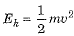Example: An object of mass 15 kg is moving with a uniform velocity of 4 m s–1. What is the kinetic energy possessed by the object?

Solution: The kinetic energy (KE) possessed by an object can be calculated using the formula:

KE = (1/2) mv2

where:

• KE is the kinetic energy
• m is the mass of the object
• v is the velocity of the object

In this case, the mass of the object is given as 15 kg and the velocity is given as 4 m/s. Plugging these values into the formula, we can calculate the kinetic energy:

KE = (1/2) * 15 kg * (4 m/s)2

= 0.5 * 15 kg * 16 m2/s2

= 120 Joules (J)

Therefore, the object possesses a kinetic energy of 120 Joules.

### Potential Energy

Potential energy possessed by an object is the energy present in it by virtue of its position or configuration (i.e., size and shape) or change thereof.
Water stored in a dam, a stretched or compressed spring, stretched bow and arrow, an object situated at a height possess potential energy.

• Gravitational Potential Energy: or (potential energy (PE) of an object at a certain height) It is the simplest form of potential energy. The gravitational potential energy of an object at a point above the ground is defined as the work done in raising it from the ground to that point against gravity.
If an object of mass m is situated at a height h above the ground, then its gravitational potential energy is given by
Ep = mgh.

Example: An object with a mass of 2 kilograms is lifted to a height of 10 meters. What is its gravitational potential energy?
Solution:

We can use the equation Ep = mgh, where:

• Ep represents the gravitational potential energy,
• m represents the mass of the object,
• g represents the acceleration due to gravity,
• h represents the height.

Given:

m = 2 kilograms

g = 9.8 m/s^2 (approximate value for acceleration due to gravity on Earth)

h = 10 meters

Plugging these values into the equation, we have:

Ep = 2 kg x 9.8 m/s^2 x 10 m

Ep = 196 Joules

Therefore, the gravitational potential energy of the object is 196 Joules.

• Elastic Potential Energy: The potential energy acquired by an object due to change in its configuration is known as the ‘elastic potential energy’.
Change in potential energy between two given points (or the work done for taking an object from one point to another point) simply depends on the difference in vertical heights of the initial and final positions of the object and does not depend on the path along which the object is moved.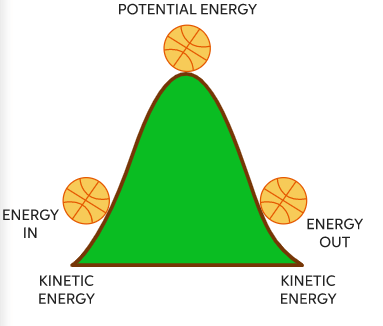Conversion of Kinetic energy to Potential energyIn the above diagram, a ball is at the top and bottom of a slope. When the ball is at the top, it has a high potential energy (PE) due to its position and the gravitational force acting on it. As the ball rolls down the slope, it gains speed, and its potential energy is converted into kinetic energy (KE), which is the energy of its motion.

Question for Chapter Notes: Work and Energy
Try yourself:Question 2: Water stored in a dam possesses

### Law of Conservation of Energy

According to the law of conservation (transformation) of energy, we can neither create nor destroy energy. Energy may only be transformed from one form to another such that total energy before and after the transformation remains exactly the same.

• Energy can be changed from one form to another. But the total energy remains unchanged. Energy lost in one form exactly reappears in some other form so that total energy remains unchanged.
• The law of conservation of energy is valid in all situations and for all sorts of transformations. Thus, like conservation law of momentum, the conservation law of energy too is a fundamental law of nature that never fails.
• For a freely falling object, there is a continual transformation of gravitational potential energy into kinetic energy such that their sum remains constant at all points during the fall.

Example of Law of conservation of Energy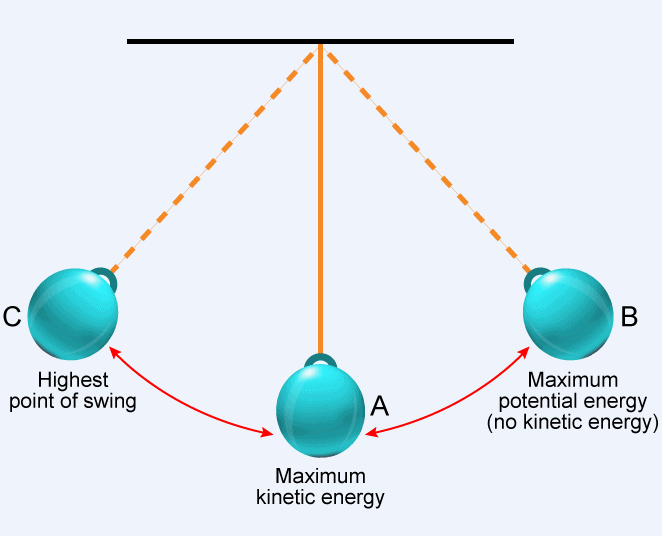A Pendulum

The above diagram shows a pendulum, which is consists of a mass (m) connected to a fixed pivot point via a string of length (L).

Here's a description of the pendulum at different positions to explain the law of conservation of energy:
1. At the highest point (A): At this point, the pendulum is momentarily at rest and all its energy is potential energy (PE). The height (h) of the mass above the lowest point determines the amount of potential energy. PE = m * g * h, where g is the acceleration due to gravity.
2. At the lowest point (B): As the pendulum swings down, its potential energy is converted into kinetic energy (KE). At the lowest point, its height (h) is zero, so it has no potential energy. At this point, all its energy is kinetic energy, and the pendulum is moving at its highest velocity. KE = 0.5 * m * v^2, where v is the velocity of the mass.
3. At the highest point on the other side (C): As the pendulum swings upward, its kinetic energy is converted back into potential energy.

## Rate of doing Work or Power

The rate of doing work or the rate of transfer of energy is known as the power.
∴ Power = work / time
=> P = W/t

• Power means speed with which a machine does work or the speed at which a machine supplies energy.
• SI unit of power is a watt. Power is said to be 1 watt (1 W) if rate of doing work is 1 Js-1.
• Some other common units of power are:
1 kilowatt = 1 kW = 1000 W = 1000 J s-1
1 megawatt = 1 MW - 106 W - 106 J s-1
1 horse power = 1 hp = 746 watt.

Question for Chapter Notes: Work and Energy
Try yourself:A machine does 18000 Joules of work in 3 minutes. What is the average power of the machine in watts?

### Average Power

If power of a person/machine varies with time, then his average power may be obtained by dividing the total energy consumed (or total work done) by the total time taken.
Average Power = Total energy consumed(or total work done) / Total time

### Commercial Unit of Power

• For electrical energy used in households and commercial/industrial establishments we generally use a bigger unit of energy, which is known as 1 kilowatt hour (1 kW h).
• One kW h is the total energy consumed in 1 hour at the rate of 1 kW (or 1000 W or 1000 J s-1).
1 kWh = 3.6 x 106 J
The document Work and Energy Chapter Notes | Science Class 9 is a part of the Class 9 Course Science Class 9.
All you need of Class 9 at this link: Class 9

## Science Class 9

66 videos|352 docs|97 tests

## Science Class 9

66 videos|352 docs|97 testsExplore Courses for Class 9 examSignup to see your scores go up within 7 days! Learn & Practice with 1000+ FREE Notes, Videos & Tests.
10M+ students study on EduRev
Track your progress, build streaks, highlight & save important lessons and more!
Related Searches

,

,

,

,

,

,

,

,

,

,

,

,

,

,

,

,

,

,

,

,

,

;